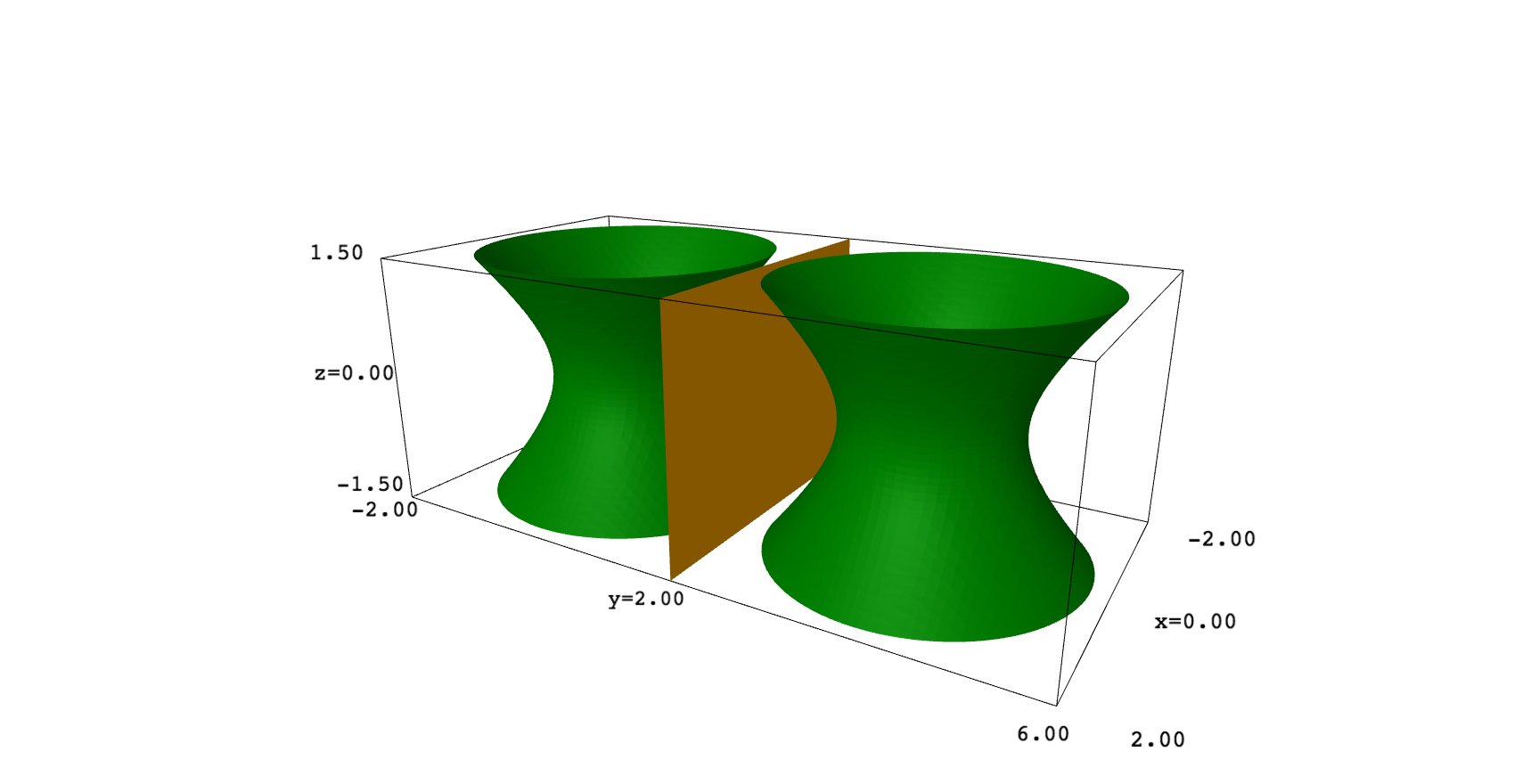I don't understand what it is $R^{(-1,1)}$ and, IMHO, an hyperboloid is a surface, so it should be traced in 3D. In such a case, you can play with translations, scales and rotations to apply reflections. For example, this code plots a one sheet hyperboloid and mirrors it on the plane $y=2$:
var("x,y,z")